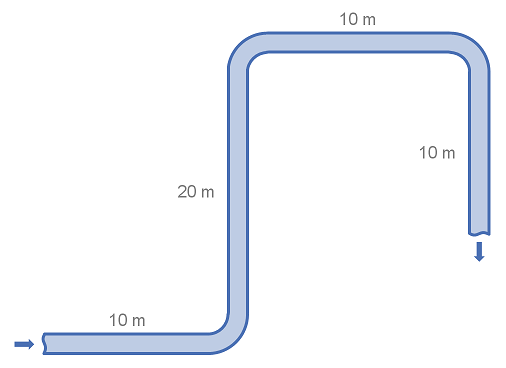# Summary

Fittings such as elbows, tees, valves and reducers represent a significant component of the pressure loss in most pipe systems. This article details the calculation of pressure losses through pipe fittings and some minor equipment using the 3K method.

# Definitions

 : Internal diameter of pipe : nominal diameter of pipe in inches : Resistance Coefficient : 3K method constant : 3K method constant : 3K method constant : Reynolds number

# Introduction

The 3K method allows the user to characterise the pressure loss for flow through fittings in a pipe. As the name suggests, three K coefficients are used to characterise the fitting, which when combined with the flow conditions and the pipe diameter may be used to calculate the fitting K value. Once the K value has been determined the head or pressure loss through the fitting may be calculated.

Like the 2K method, the 3K method is an improvement on the K value (excess head) method. While the 2K method improves on the excess head method by characterising the change in pressure loss due to varying Reynolds Number the 3K method further improves the accuracy of the pressure drop prediction by also characterising the change in geometric proportions of a fitting as its size changes. This makes the 3K method particularly accurate for a system with large (greater than DN300/12in) fittings.

For alternative methods of characterising pressure loss for flow through fittings and a discussion of which method is most appropriate please refer to the summary of methods for estimating pressure loss from fittings.

# Calculation of K value using the 3K Method

The 3K method is an extension of the 2K method and therefore the Excess Head (K method). As such the 3K method requires the 3K coefficients to be converted into a K value before the fitting pressure drop may be calculated.

## Formula for Calculating the K Value from the 3K Method

The K value may be calculated from the 3K coefficients as demonstrated in the equation below.

As you can see from the equation above the calculation of the K value depends on both the fluid Reynolds number and the size of the fitting

## Table of 3K Coefficients

The table below contains the 3K parameters for a wide range of commonly encountered fittings

FittingTypes(in0.3)(mm0.3)
90° Elbow, Flanged or WeldedStandard Radius (R/D = 1)8000.091410.6
90° Elbow, Mitered1 weld 90°10000.27410.6
2 welds 45°8000.0684.110.8
3 welds 30°8000.0354.211.1
45° Elbow, Mitered1 weld 45°5000.086410.6
2 welds 22.5°5000.052410.6
180° Bendthreaded, close-return (R/D = 1)10000.23410.6
flanged (R/D = 1)10000.12410.6
all types (R/D = 1.5)10000.1410.6
Tee Through-branch as an Elbowthreaded (r/D = 1)5000.274410.6
flanged (r/D = 1)8000.28410.6
stub-in branch10000.34410.6
flanged (r/D = 1)1500.05410.6
stub-in branch100000
Angle valve45°, full line size, = 19500.25410.6
90° full line size, = 110000.69410.6
Globe valvestandard, = 115001.73.69.5
Plug valvebranch flow5000.41410.6
straight through3000.0843.910.3
three-way (flow through)3000.14410.6
Gate valvestandard, = 13000.0373.910.3
Ball valvestandard, = 13000.0173.59.2
Diaphragmdam type10000.694.912.9
Swing check valveVmin = 35 15000.46410.6
Lift check valveVmin = 40 20002.853.810

# Example Calculation of Pressure Loss using 3K-values

This example demonstrates how to use the 3K method to calculate the head loss through simple pipe and fitting arrangement. The example uses water in system of standard weight carbon steel pipe. Note that for this example we consider a flat system, with no elevation changes.## System details

 Pipe Size : DN100 (4") Pipe Diameter (Nominal) : 4" Pipe Internal Diameter : 102.3 mm Pipe Length : 50 m Fittings : 3 x 90° long radius (R/D = 2) flanged elbows Fluid Velocity : 3 m/s Fluid Density : 1000 kg/m3 Reynolds Number : 306,900 Friction Factor : 0.018 Gravitational Acceleration : 9.81 m/s2

## Calculation

Using the table of fittings and 3K-values above we find that the excess head or K-value for the 90° elbow.

With this we can calculate the head loss for a single elbow.

Using the equation for head loss in pipe, we can calculate the loss through the straight piping:

The total head loss for the system is the addition of the head loss from the pipe and the fittings.

In the above example we first calculated the head loss for a single fitting and then multiplied by the number of fittings. It is also correct to add or multiply the K-values of fittings and then covert to a pressure or head loss. This is useful when there are many fittings of several types. However the 3K values must be converted to single K values for each fitting type individually.

Article Tags

# Subscribe to our mailing list

 Email Name